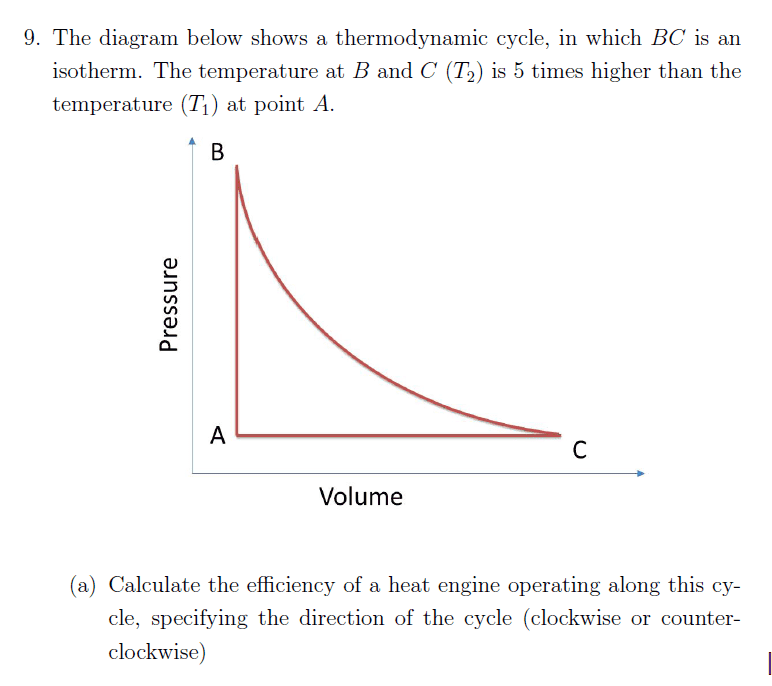# Efficiency of a heat engine

## Homework Statement

I am having an issue trying to decipher this question, as I am not sure if it a lack of knowledge on my half or there is an assumption I have to make.## Homework Equations

##\epsilon=\frac{W_{total}}{Q_{in}}##

## The Attempt at a Solution

My issue is calculating the heat along the path ##Q_{A \rightarrow B}## my confusion stem from that second law there is no working being done so then heat is equal to ##Q_{A \rightarrow B}=C_v\Delta T##.

Now in the question I have no information on if this a monotonic gas or diatomic ideal gas, if I have to make the assumption it is fine I can calculate the efficiency just fine, but I was just wondering if someone could take a look as I may have overlooked something.

#### Attachments

Chestermiller
Mentor
Just do it algebraically, and see what the efficiency looks like.

Also, does 5x higher mean 5x as high, or does it mean 6x as high?

Last edited:
I have attempted to solve algebraically and seem to have gotten a result which, dose not simplify easily.

Here is my current working

Step 1 : What I know.

$$\Delta E_{int}=Q_in+Wd_{on} \ $$

$$\Delta E_{int}=C_v n\Delta T \ $$

$$\Delta Wd_{on}=-\Delta Wd_{by}=-P\Delta V \ $$

In the PV diagram displayed I have three processes
1. Isotherm path ##B \rightarrow C##
2. Isobaric path ##C \rightarrow A##
3. Isochoric paths ##A \rightarrow B##
Step 2 : Solving Isotherm path
$$\Delta E_{int}=0$$

$$0=Q_{in}+Wd_{on}=Q_{in}-Wd_{by}$$

$$Q_{in}=Wd_{by}=Pdv$$

$$P=\frac{nR5T_1}{V}$$

$$Qin=\int^{V_2}_{V_1} \frac{nR5T_1}{V} dv=nR5T_1 ln(\frac{V_2}{V_1})$$

finding ##\frac{V_2}{V_1}##

$$P_1V_1=nRT_1$$

$$PV_2=nR5T_1$$

$$\frac{V_2}{V_1}=5$$

therefore

$$Q_{in}=nR5ln(5)T_1=Q_H$$

Step 3 : Solving Isobaric path

$$\Delta E_{int}=Q_{in}-Wd_{by}$$

$$C_vn\Delta T=Q_{in}-P(\Delta T)$$

$$Q_{in}=C_vn\Delta T + nR\Delta T$$

$$(C_v+R)n\Delta T=Q_{in}$$

$$C_p n\Delta T = Q_{in}$$

$$\Delta T=-4T_1$$

$$Q_{in}=-4C_pnT_1$$

Step 4 : Solving Isobaric path

$$\Delta E_{int}=Q_{in}$$

$$C_v n \Delta T=Q_{in}$$

$$4C_v n T_1=Q_{in}$$

Step 5 : Solving for Efficiency

$$\epsilon = \frac{W}{Q_in}$$

As system is a complet cycle then ##\Delta E_{int}=0## therefore ##W_T=\sum Q_in##

$$\epsilon = \frac{(5ln5)R+4C_v-4C_p}{(5ln5)R+4C_v}$$

$$\frac{(5ln5)R-4R}{(5ln5)R+4C_v}=\frac{(5ln5-4)R}{(5ln5)R+4C_v}$$

And this is were I am stuck, I have I missed a step in my workings? Surley I should be able to simplyfy this in to an actual percentage

Chestermiller
Mentor
You almost have it.

$$\frac{(5ln5)R-4R}{(5ln5)R+4C_v}=\frac{(\ln{5}-0.8)R}{(\ln{5}R+0.8C_v}=\frac{\left(1-\frac{0.8}{\ln{5}}\right)}{\left(1+\frac{0.8}{\ln{5}(\gamma-1)}\right)}$$

•Taylor_1989